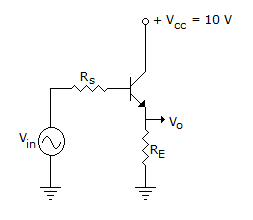# Electronics and Communication Engineering - Analog Electronics

26.

Which of the following?A. Voltage series feedback and decrease I/P impedance. B. Voltage shunt feedback and decrease I/P impedance. C. Voltage series feedback and increase I/P impedance. D. Voltage shunt feedback and increase I/P impedance.

Explanation:

Because sample signal is voltage and feedback signal is also voltage. The drop in RE change the input voltage at Base Emitter Junction.

27.

In a transistor E mode the collector current with the transistor in cut off region is

 A. zero B. high C. equal to saturated value D. none of the above

Explanation:

When transistor is cut off, IC is zero.

28.

Two CE stages, 1 and 2 are coupled through a capacitor. VCC is the same for both. Base resistances RB1 and RB2 are such that RB1 > RB2. Then

 A. base currents for both stages are equal B. base current of stage 1 is more than that of stage 2 C. base current of stage 2 is more than that of stage 1 D. either (b) or (c)

Explanation:

Since RB2 < RB1, IB2 > IB1.

29.

In a two stage CE amplifier circuit, the ac collector resistance of the first stage depends on

 A. load resistance B. input impedance of first stage C. input impedance of second stage D. all of the above

Explanation:

Since the first amplifier is feeding the second stage, the input impedance of second stage is the ac collector resistance of first stage.

30.

In a CE amplifier the ac cut off voltage is 9 V and slope of ac load line is - 0.5 mA/V. The ac saturation current is

 A. 9 mA B. 4.5 mA C. 3 mA D. 18 mA